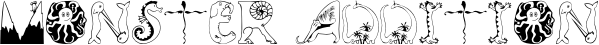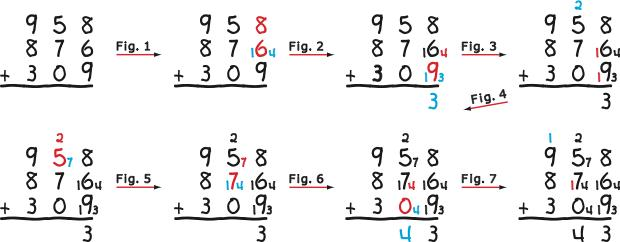One of the first tasks in elementary school math is to learn the addition facts: 1+2=3, 3+4=7, 5+6=11 and the like. There are 100 of them, from 0+0=0 to 9+9=18, and children are given the first 2-3 years in school to learn them, which basically means to memorize them to where they can recite them without any evidence of thinking such as counting them out on their fingers. Stories of being smacked on the knuckles by a ruler while figuring out an addition fact are common. Even if memorized perfectly, though, knowing the number facts is of limited value relative to the standard addition algorithm.

Note what occurs in adding three or more numbers at a time. In the problem below, beginning with the unit’s column, 8+6=14 and 14+9=WHAT!

9 5 8

8 7 6

+ 3 0 9

Where did 14+9 come from? It is not an addition fact! It has not been drummed in with flash cards and speed drills. So out come the fingers or, safer, sticks drawn off to the side of the problem that are counted and then erased. Either that or guessing. It is the same throughout the problem. This is why the textbooks and worksheets for math in the early grades do not require children to add more than two numbers at a time unless the numbers are so small that they do not result in a sum that is not a number fact. The net effect of such coddling is to teach “always carry a 1 as addressed elsewhere in this website.

Monster Addition eliminates the need to restrict the size of addition problems for young children. It reduces any addition problem—no matter how huge—to adding just two single-digit numbers at a time, so it relies solely on knowing only the number facts. (Another name for the algorithm is the Addition Facts Algorithm. For a variation of the algorithm, see .) Moreover, it is a reflection of importing in Ten Land on a Fair Lands™ activity board, so it is sensible and easy to teach and learn. The following illustrates its use to solve the “mini-monster problem above.CONTINUED: Next > | 1 | 2 |